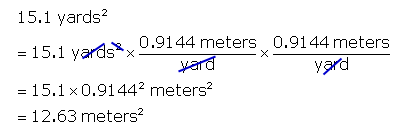SEARCH HOMEMath Central Quandaries & QueriesQuestion from Andrew, a student: If a lawn is 95.3 square yards, how do I convert that to square meters?Hi Andrew.

I'll show you how to do it for 15.1 square yards.  First you need to know that 1 yard equals 0.9144 meters.  This means we can multiply anything by (0.9144 meters / 1 yard) because the numerator and
denominator are the same, so we are multiplying by 1.  That means when we multiply anything by (0.9144 meters / 1 yard), we're really just changing the units.Hope this helps,
Stephen La Rocque.Math Central is supported by the University of Regina and The Pacific Institute for the Mathematical Sciences.